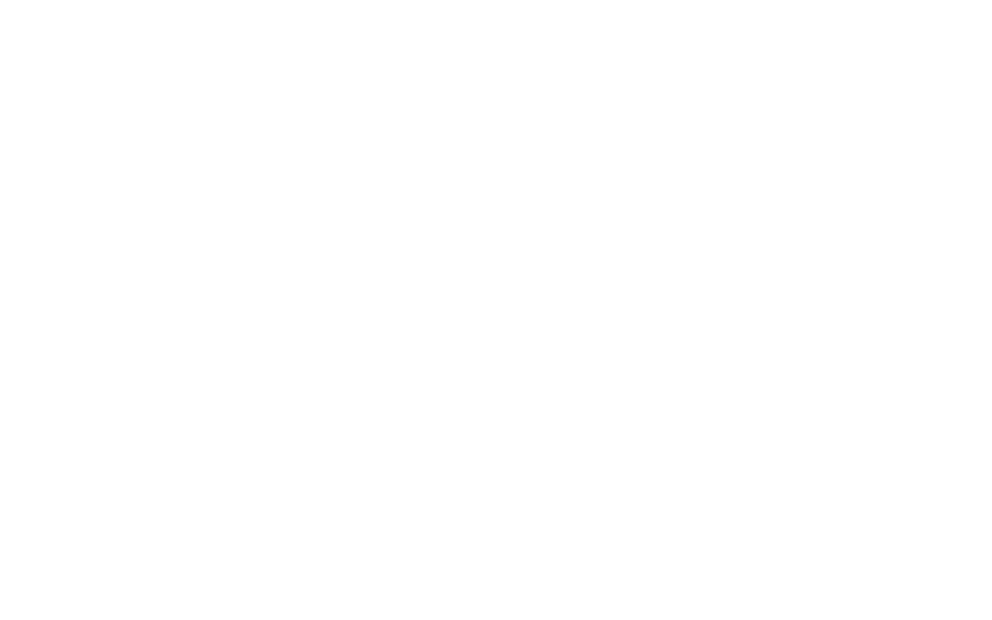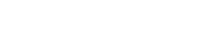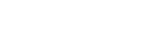# Deep Learning Neural Networks – PART TWO

Jasmin Kurtanović, Software Engineer
17.11.2020.## Creating a Simple Deep Classification Network

Based on convolutional neural network architecture, which we explained in part one of this article series, we will show you how to create a simple deep learning convolutional neural network for image classification for the CIFAR-10 dataset.

## Preparation For Model Training

The CIFAR-10 dataset consists of 60.000 32×32 color images in 10 classes, with 6000 images per class. There are 50.000 training images and 10.000 test images.

The dataset is divided into five training batches and one test batch, each with 10.000 images. The test batch contains exactly 1000 randomly selected images from each class. The training batches contain the remaining images in random order, but some training batches may contain more images from one class than another. Between them, the training batches contain exactly 5000 images from each class.

Here are the classes in the dataset, as well as 10 random images from each:

It is necessary to data process:

Separating data into training and validation sets is an important part of evaluating data mining models. The set is divided into a training and a test set (in a 80:20 ratio), so that each category has 40.000 samples for training, and the validation set contains the rest of the images from a particular category.

Building the Network

The convolutional layer is perhaps the key layer of the deep neural network because it serves to extract the characteristics of the data. Specifically, in the case of an image, the height, width and number of channels are of importance. In this case, the dimensions are 32x32x3. So, three channels are defined at the input, and 32 at the output. The next parameter is the filter size. In this case, the filter size is set to 3x3 pixels. This is followed by a layer to normalize the activations and gradients propagated through the network, thus making the network optimal for training. This is usually followed by a layer representing a nonlinear activation function. The most common activation function is the ReLU (Rectified Linear Unit) function. The final layer is a completely connected layer. In this case, three linear layers are defined. The first parameter is the size of the input, while the second parameter is the size of the output. The output size of this deep neural network is 10, because there are 10 classes that can be detected.

## Training and Evaluation

Before we train the model, we have to first create an instance of our CNN class, and define our loss function and optimizer. We use a cross entropy loss, with a momentum-based SGD or Adam optimization algorithm.

The number of epochs is looped over, and within this loop, we iterate over trainloader using enumerate, which can be seen through the train_val function.

The next step is to pass the model outputs and the true image labels to our CrossEntropyLoss function, defined as criterion. The loss is appended to a list that will be used later to plot the progress of the training. The next step is to perform back-propagation and an optimized training step. First, the gradients have to be zeroed, which can be done easily by calling zero_grad() on the optimizer. Next, we loss .backward() on the loss variable to perform the back-propagation. Finally, now that the gradients have been calculated in the back-propagation, we simply call optimizer.step() to perform the SGD/Adam optimizer training step.

The monitoring of the accuracy and loss during training is shown in the following figures:

Summary

In these two posts, the goal was to explain the main concepts behind Convolutional Neural Networks in simple terms. CNN is a neural network with several convolutional and several other layers. The convolutional layer has a number of filters that perform a convolutional operation. The process of building a CNN always involves four major steps –  Convolution, Pooling, Flattening and Full connection, which was covered in detail. Choosing parameters, applying filters with strides, padding if required. Performing convolution on the image and applying ReLU activation to the matrix is the main core process in CNN and if you get this incorrect, the whole joy is over then and there.

By understanding the theory of neural networks and their nature, together with their origin and subject of inspiration, it can be concluded that this is definitely one of the technologies of the future, which will eventually permeate almost all aspects of human activity.